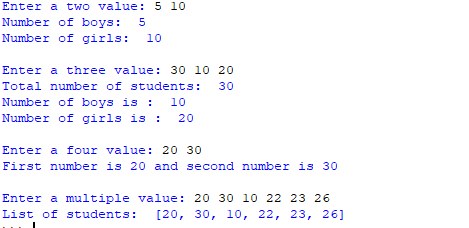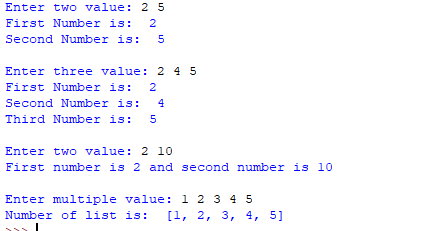# Python 教程之输入输出（3）—— 在 Python 中从用户那里获取多个输入

• 2022 年 8 月 03 日
• 本文字数：1126 字

阅读完需：约 4 分钟

• 使用 split() 方法

• 使用列表理解

input().split(separator, maxsplit)

x, y = input("Enter two values: ").split()print("Number of boys: ", x)print("Number of girls: ", y)print()

x, y, z = input("Enter three values: ").split()print("Total number of students: ", x)print("Number of boys is : ", y)print("Number of girls is : ", z)print()

a, b = input("Enter two values: ").split()print("First number is {} and second number is {}".format(a, b))print()

# and type casting using list() functionx = list(map(int, input("Enter multiple values: ").split()))print("List of students: ", x)x, y = [int(x) for x in input("Enter two values: ").split()]print("First Number is: ", x)print("Second Number is: ", y)print()

x, y, z = [int(x) for x in input("Enter three values: ").split()]print("First Number is: ", x)print("Second Number is: ", y)print("Third Number is: ", z)print()

x, y = [int(x) for x in input("Enter two values: ").split()]print("First number is {} and second number is {}".format(x, y))print()

x = [int(x) for x in input("Enter multiple values: ").split()]print("Number of list is: ", x)# 一次接受多个输入，用逗号分隔x = [int(x) for x in input("Enter multiple value: ").split(",")]print("Number of list is: ", x)### 海拥(haiyong.site)

【个人网站】haiyong.site 【联系方式】微信：wh18363 【软件技能】前端，Java，Python 【个人称号】InfoQ 写作社区签约作者，华为云享专家，CSDN原力作者，全栈领域优质创作者，掘金2021年度人气作者No.21

## 评论